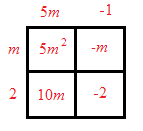### Home > CCA2 > Chapter Ch3 > Lesson 3.2.1 > Problem3-69

3-69.

Multiply the expressions below using generic rectangles. Homework Help ✎

1. $(5m−1)(m+2)$$5m^2+9m−2$

1. $(6−x)(2+x)$

Refer to part (a).

1. $(5x−y)^2$

Refer to part (a).

1. $3x(2x−5y+4)$

Refer to part (a).